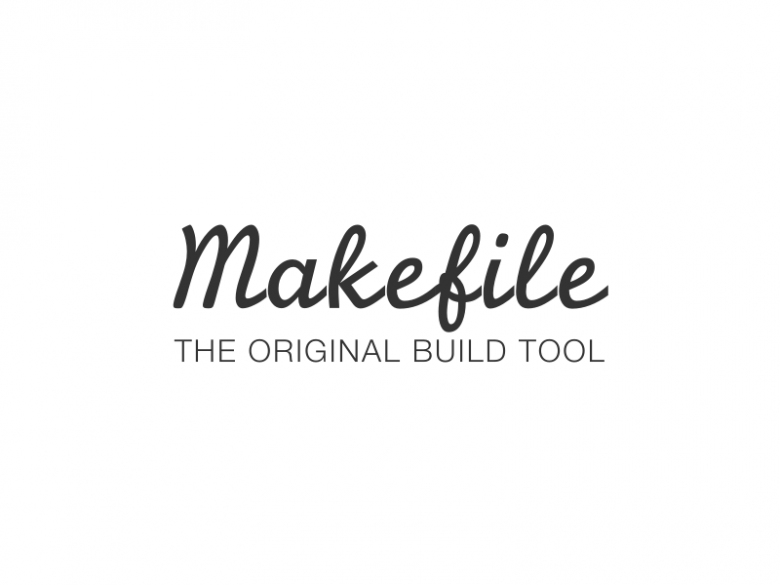# C/C++ 项目构建指南：如何使用 Makefile 提高开发效率

• 2023-05-23
广东
• 本文字数：1220 字

阅读完需：约 4 分钟Makefile 是一个常用的自动化构建工具，它可以为开发人员提供方便的项目构建方式。在 C/C++项目中，Makefile 可以用来编译、链接和生成可执行文件。使用 Makefile 的好处是可以自动执行一系列命令，从而减少手动操作的复杂性和出错的可能性。此外，Makefile 还可以根据源代码的修改情况，自动更新目标文件，从而提高了构建的效率和准确性

## Makefile 的基础知识

Makefile 由一系列规则组成。每个规则包括一个目标(target)、一个或多个依赖(dependencies)和一组命令(commands)。目标是我们想要生成的文件，依赖是生成目标所需要的文件，命令是生成目标的具体步骤。

target: dependencies    commands

## 使用 Makefile 构建 C/C++项目

CC = gccCXX = g++CFLAGS = -Wall -O2CXXFLAGS = -Wall -O2SRCDIR = srcOBJDIR = objBINDIR = binSRC = $(wildcard$(SRCDIR)/*.c $(SRCDIR)/*.cpp)OBJ =$(patsubst $(SRCDIR)/%.c,$(OBJDIR)/%.o,$(filter %.c,$(SRC))) \\      $(patsubst$(SRCDIR)/%.cpp,$(OBJDIR)/%.o,$(filter %.cpp,$(SRC)))BIN =$(BINDIR)/myapp$(BIN):$(OBJ)    $(CXX)$(CXXFLAGS) -o $@$^$(OBJDIR)/%.o:$(SRCDIR)/%.c    $(CC)$(CFLAGS) -c -o $@$<$(OBJDIR)/%.o:$(SRCDIR)/%.cpp    $(CXX)$(CXXFLAGS) -c -o $@$<

## 总结

• 静态代码分析工具可以帮助检测代码中的错误和漏洞。

• 自动化测试工具可以帮助自动化测试过程，提高测试效率。

• 持续集成工具可以帮助自动化构建、测试和部署过程，提高开发效率。## 评论